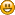Hello Guest

# 3D movement calculation

• 2 Replies
• 9734 Views####countnoobula

•• 3##### 3D movement calculation
« on: July 18, 2013, 13:26:02 »
Hi Guys,

New here, well, lurk a lot, don't post much. A bit shyAnyway, I am having some trouble with calculations with moving my objects in a 3d space.

The exact object in question is a bullet. If I click, I need it to follow the path until either it exits the map or similar, but the part I am struggling with is setting the speed along the X axis, speed along the Y axis, and speed along the Z axis.

To elaborate further, when the user fires, it must spawn the entity and send it travelling in that direction at it's preset speed.

I hope this makes sense and that you can help please. I am not that great at Trig so have been failing at getting the speed it must go at.####ic5y

•• 19##### Re: 3D movement calculation
« Reply #1 on: July 23, 2013, 09:31:11 »
What I would do, is calculate the direction from the camera's orientation.

I have values for rotation (Vector3f, so rotation around X is rotation.getX()). then direction.x = Math.cos(Math.toRadians(rotation.getY() + 90)); <- because of OpenGL's 0 rotation means forward, if you see the Y axis. If your rotation around Y is 0, you are facing -Z direction.

then direction.z = Math.sin(Math.toRadians(rotation.getY() + 90);

and direction.y = Math.cos(Math.toRadians(rotation.getX() + 90);

I'm not sure if this works. Try to visualize this on paper, it usually helps me####countnoobula

•• 3##### Re: 3D movement calculation
« Reply #2 on: August 15, 2013, 07:10:38 »
ThanksSorry for late reply, it worked out thoughGot it working very nicely